Get instant live expert help with Excel or Google Sheets“My Excelchat expert helped me in less than 20 minutes, saving me what would have been 5 hours of work!”

#### Post your problem and you'll get expert help in seconds

Your message must be at least 40 characters
Our professional experts are available now. Your privacy is guaranteed.

# Learn from a Risk Matrix Example in Excel

We can apply a risk matrix to a set of data to determine the risk that a hazard poses. We can do this with the INDEX and MATCH function. In this Matrix, the Y-axis tells us the SEVERITY of the risk involved and the X-axis tells us the LIKELIHOOD of occurrence of the RISK. Hence, the RISK of a hazard is calculated as:

`Risk of hazard = likelihood of occurrence (probability) * Severity of harm`

We will walk through the steps below to understand the process.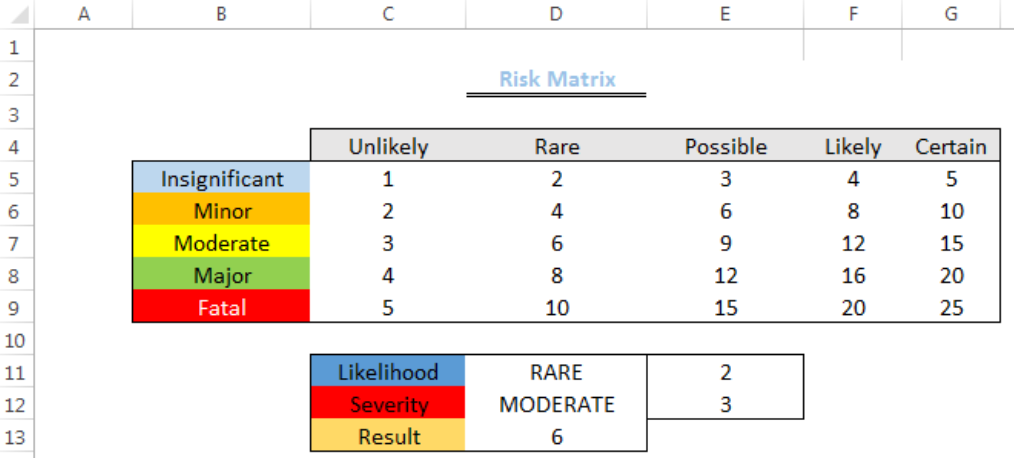Figure 1: How to Use a Risk Matrix

## Formula

The formula in Cell D13 is given as:

`=INDEX(C5:G9,MATCH(Severity,B5:B9,0),MATCH(Likelihood,C4:G4,0))`

## Setting up the Data

We will set up the risk matrix by doing the following:

• We will type the severity on the Y-axis (Cell B5 to Cell B9)
• We will type the likelihood on the X-axis (Cell C4 to Cell G4)
• We will input the numbers as shown in figure 2 in the range of C5:G9
• Cell C11, C12, and C13 will be named likelihood, severity, and result respectively
• Cell D11 and D12 will be named as RARE and MODERATE respectively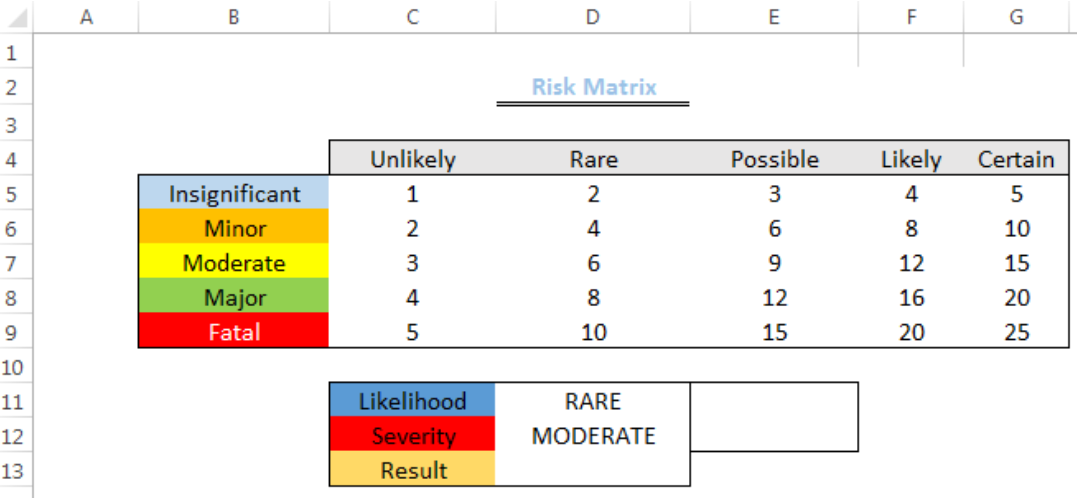Figure 2: Setting up the Risk Matrix Data

## Determining the Risk

• We will click on Cell E11
• We will type in this string: `=MATCH(D11,C4:G4,0)`
• Click on Enter
• Likewise, we will click on Cell E12
• We will type in this string: `=MATCH(D12,B5:B9,0)`
• We will click on Enter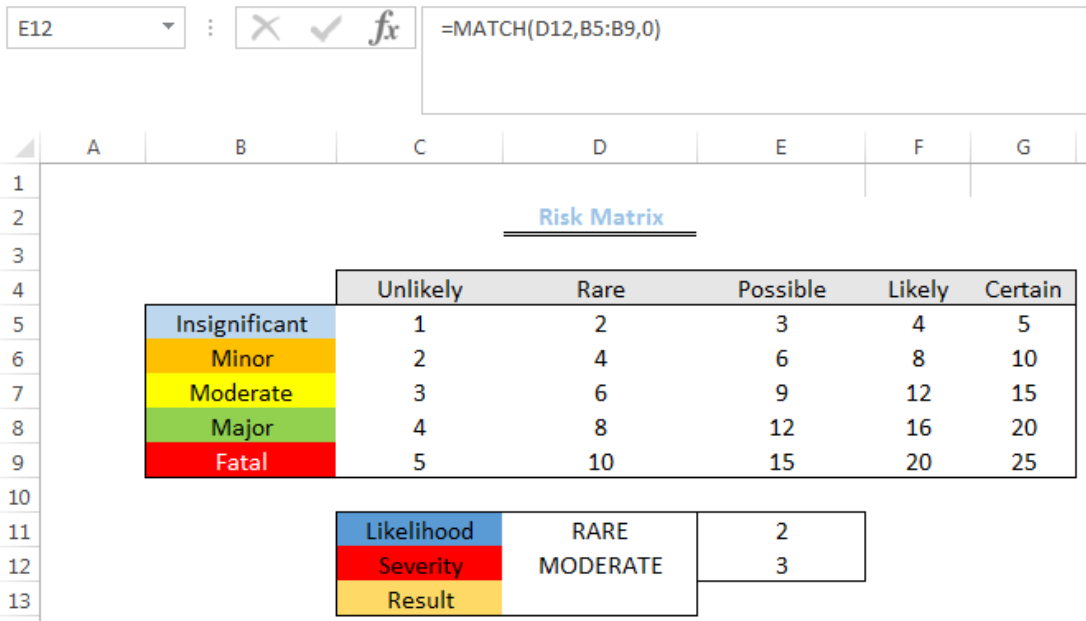Figure 3: Determining the Result of the Inputted Data in the Risk Matrix

We will type the formula below into Cell D13

`=INDEX(C5:G9,MATCH(D12,B5:B9,0),MATCH(D11,C4:G4,0))`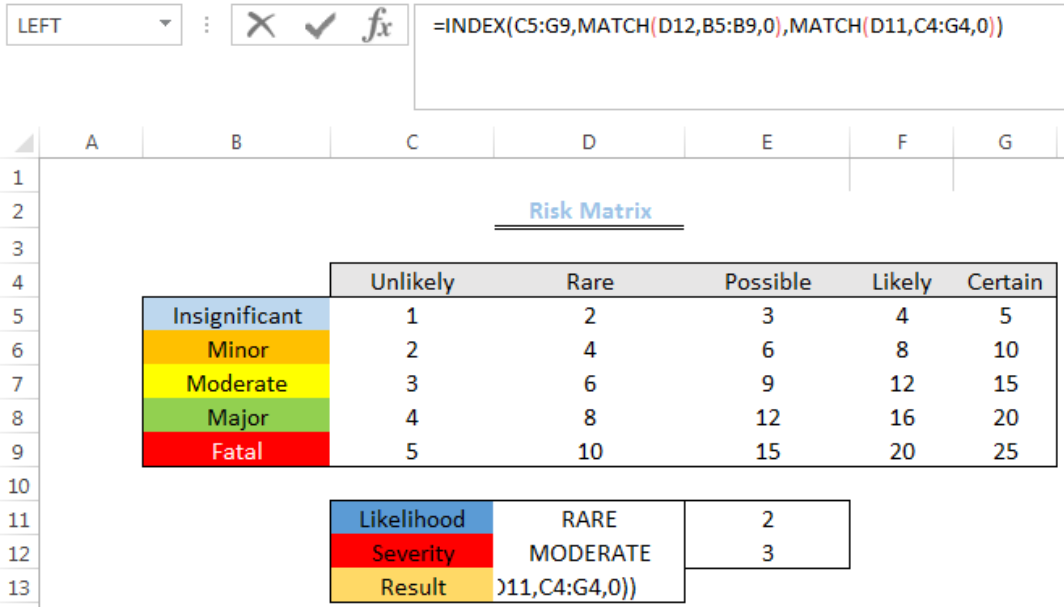Figure 4: Determining the Result of the Inputted Data in the Risk Matrix

We will press enter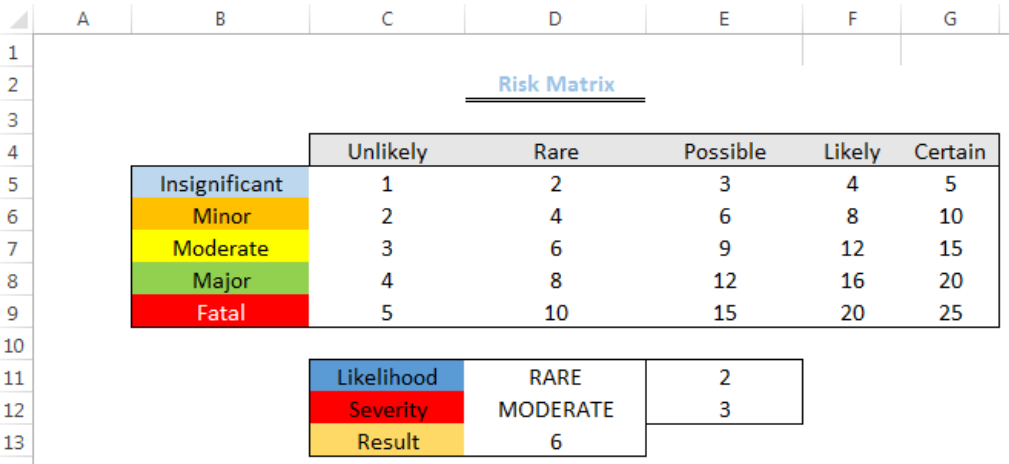Figure 5: Result of the Inputted Data in the Risk Matrix

## Explanation

• MATCH function

The match function matches the lookup value within a specified range and we have set it to return an exact match. For instance, when determining the likelihood in the example, we typed in the string below:

`=MATCH(D11,C4:G4,0)`

D11 is the lookup value, C4:G4 is the range and zero indicates an exact match.

The same scenario applies to determine the severity.

• INDEX function

The INDEX function returns the number or value that is present in ROW 3 and COLUMN 2 based on this string: `=INDEX(C5:G9,E11,E12)`

This method is preferred to the ALTERNATIVE METHOD because we can vary the SEVERITY and LIKELIHOOD in Cells D11 and D12 respectively to AUTOMATICALLY get the result.

## Alternative method

• We will click on Cell D13
• We will type the string below into the Cell
=INDEX(C5:G9,MATCH(D12,B5:B9,0),MATCH(D11,C4:G4,0))
• We will click on EnterFigure 6: Result of the Alternative method of applying the Risk Matrix

## Instant Connection to an Expert through our Excelchat Service

Most of the time, the problem you will need to solve will be more complex than a simple application of a formula or function. If you want to save hours of research and frustration, try our live Excelchat service! Our Excel Experts are available 24/7 to answer any Excel question you may have. We guarantee a connection within 30 seconds and a customized solution within 20 minutes.

### Did this post not answer your question? Get a solution from connecting with the expert.Another blog reader asked this question today on Excelchat:## Subscribe to Excelchat.coAnother blog reader asked this question today on Excelchat: